## The equation of state of a perfect gas:

Gas laws are study of any two of quantities like pressure, volume and temperature when the third is kept constant

## Boyle’s Law

It states that the volume of a given mass of a gas is inversely proportional to its pressure, provided the temperature remains constant.

Mathematically,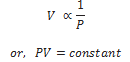If P1 , V1 are initial pressure and volumes and P2 , V2 be final values then,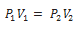Graph between P and V at temperature T1 and T2 such that T1 < T2 are shown below,Graph above shows that Boyle’s law is strictly not obeyed by gases at all values of P and T but it obeys this law only at low pressure and high temperature i.e., at law density

## Charles’s Law

It states that if the pressure remains constant, then the volume of a given mass of gas increases or decreases by 1/273.15 of its volume at 0℃ for each 1℃ rise or fall of temperature.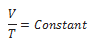### Graph between V and T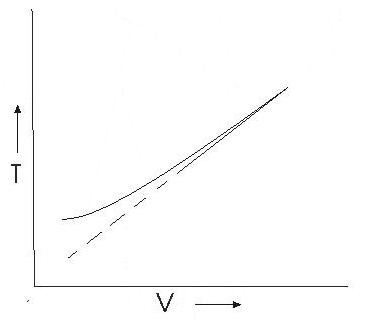This graph shows that experimental graph deviates from a straight line. Theoretical and experimental graphs are in agreement at high temperature.

## Ideal Gas Equation

The ideal gas equation connecting pressure (P), volume (V) and absolute temperature (T) is

PV = Constant

PV = μ RT = kB NT

where μ is the number of moles and N is the number of molecules. R and kB are universal constants.

R = 8.314 J mol-1 K-1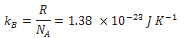Real gases satisfy the ideal gas equation only approximately, more so at low pressures and high temperatures.